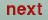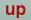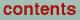Next: Band structure plot Up: Plot programs Previous: Charge density and ELF

## Hartree potential plot

The program gnupot.run generates plots of the superimposed atomic Hartree potentials of the free atoms. This plot can be useful to check the estimate of the sphere radii performed by lmhart.run. The program reads the file POT created by lmhart.run for the plane specified in the PLOT category in the CTRL file and creates the plot data file GPOT.DAT and the command file POT.GNU. After the selection of the output device the plot data are read in and the minimum and maximum are determined:

```lines:  2700
the potential range is from -.1E+7 to -.829E-1```
By default the plot range extends from the minimum to the maximum of the data. Since these potentials usually cover several orders of magnitude, it is advisable to modify the range by entering two scalars bounding the new interval (e.g. -5.0 0.0) after this prompt:
`  enter new min, max or "/" for default`
The following question concerns the number of isocontour lines to be drawn
` how many contours? (default is 11)`
You may enter any number between 2 and 98 or / to accept the default. The iso contours are now equally spaced from minimum to maximum including the upper and lower bound as explained in the gnucharge section. The next question defines the nature of the plot:
` draw a 3d-surface(t) or not(f)?(default: f)`
Entering t (logical true) places a 3d surface with hidden-line-removal on top of the contour plot, whereas f (logical false) or / lead to a simple contour line plot. Finally you are prompted for a 40-character title string for the plot:
` enter plot title :`
Now the plotfile or the plot window should be generated.
As stressed in the gnucharge section, Gnuplot may change the x-y-scaling.

O. Jepsen
Thu Oct 12 14:48:45 MESZ 2000